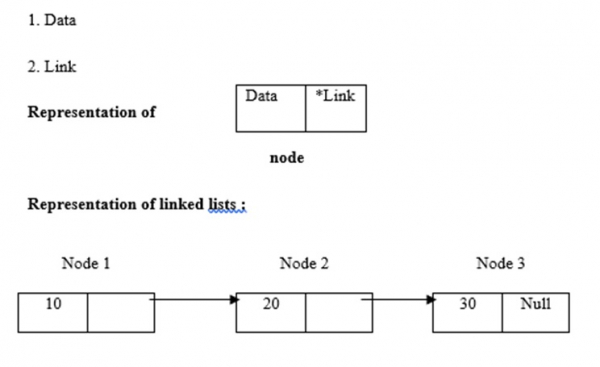# C program to find the length of linked list

CServer Side ProgrammingProgramming

Linked lists use dynamic memory allocation i.e. they grow and shrink accordingly. They are defined as a collection of nodes. Here, nodes have two parts, which are data and link. The representation of data, link and linked lists is given below −Linked lists have four types, which are as follows −

• Single / Singly linked lists
• Double / Doubly linked lists

The logic we used to find the length of linked list by using recursion method is −

int length(node *temp){
if(temp==NULL)
return l;
else{
l=l+1;
length(temp->next);
}
}

## Program

Following is the C program to find the length of linked list −

Live Demo

#include <stdio.h>
#include <stdlib.h>
int data;
}node;
int l=0;
int main(){
int len,choice,count=0,key;
do{
temp=(node *)malloc(sizeof(node));
if(temp!=NULL){
printf("\nenter the elements in a list : ");
scanf("%d",&temp->data);
temp->next=NULL;
}else{
while(temp1->next!=NULL){
temp1=temp1->next;
}
temp1->next=temp;
}
}else{
printf("\nMemory is full");
}
printf("\npress 1 to enter data into list: ");
scanf("%d",&choice);
}while(choice==1);
printf("The list has %d no of nodes",l);
return 0;
}
//recursive function to find length
int length(node *temp){
if(temp==NULL)
return l;
else{
l=l+1;
length(temp->next);
}
}

## Output

When the above program is executed, it produces the following result −

Run 1:
enter the elements in a list: 3
press 1 to enter data into list: 1
enter the elements in a list: 56
press 1 to enter data into list: 1
enter the elements in a list: 56
press 1 to enter data into list: 0
The list has 3 no of nodes
Run 2:
enter the elements in a list: 12
press 1 to enter data into list: 1
enter the elements in a list: 45
press 1 to enter data into list: 0
The list has 2 no of nodes